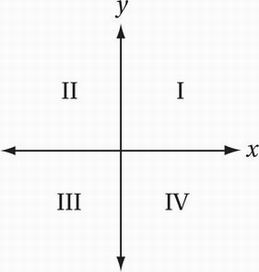# SAT Math Multiple Choice Question 741: Answer and Explanation

### Test Information

Question: 741

6.The figure above shows the locations of quadrants I-IV in the xy-plane. Which of the following represents a pair of linear equations that do NOT intersect in quadrant I?

• A. 3x + 5y = 15

y = 4

• B. 5x + 3y = 15

y = 4

• C. 5x - 3y = 15

y = 4

• D. 3x - 5y = 15

y = 4

Explanation:

A

Algebra (graphing lines) MEDIUM-HARD

In quadrant I, both the x- and y-coordinates are positive. Since y = 4 in all four systems, we simply need to find the system for which the x-coordinate of the solution is not positive. We can find the corresponding x-coordinate for each system by just substituting y = 4 and solving for x.

Substitute y = 4 into first equation in (A):

3x + 5(4) = 15

Simplify:

3x + 20 = 15

Subtract 20:

3x = -5

Divide by 3:

x = -5/3

In this case, we don't need to go any further, because the solution to the system in (A) is (-5/3, 4), which is in quadrant II, not quadrant I.